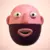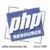## Integers

An int is a number of the set ℤ = {..., -2, -1, 0, 1, 2, ...}.

### Syntax

ints can be specified in decimal (base 10), hexadecimal (base 16), octal (base 8) or binary (base 2) notation. The negation operator can be used to denote a negative int.

To use octal notation, precede the number with a `0` (zero). As of PHP 8.1.0, octal notation can also be preceded with `0o` or `0O`. To use hexadecimal notation precede the number with `0x`. To use binary notation precede the number with `0b`.

As of PHP 7.4.0, integer literals may contain underscores (`_`) between digits, for better readability of literals. These underscores are removed by PHP's scanner.

Beispiel #1 Integer literals

``` <?php\$a = 1234; // decimal number\$a = 0123; // octal number (equivalent to 83 decimal)\$a = 0o123; // octal number (as of PHP 8.1.0)\$a = 0x1A; // hexadecimal number (equivalent to 26 decimal)\$a = 0b11111111; // binary number (equivalent to 255 decimal)\$a = 1_234_567; // decimal number (as of PHP 7.4.0)?> ```

Formally, the structure for int literals is as of PHP 7.4.0 (previously, underscores have not been allowed):

```decimal     : [1-9][0-9]*(_[0-9]+)*
| 0

octal       : 0[oO]?[0-7]+(_[0-7]+)*

binary      : 0[bB]+(_+)*

integer     : decimal
| octal
| binary
```

The size of an int is platform-dependent, although a maximum value of about two billion is the usual value (that's 32 bits signed). 64-bit platforms usually have a maximum value of about 9E18. PHP does not support unsigned ints. int size can be determined using the constant `PHP_INT_SIZE`, maximum value using the constant `PHP_INT_MAX`, and minimum value using the constant `PHP_INT_MIN`.

### Integer overflow

If PHP encounters a number beyond the bounds of the int type, it will be interpreted as a float instead. Also, an operation which results in a number beyond the bounds of the int type will return a float instead.

Beispiel #2 Integer overflow on a 32-bit system

``` <?php\$large_number = 2147483647;var_dump(\$large_number);                     // int(2147483647)\$large_number = 2147483648;var_dump(\$large_number);                     // float(2147483648)\$million = 1000000;\$large_number =  50000 * \$million;var_dump(\$large_number);                     // float(50000000000)?> ```

Beispiel #3 Integer overflow on a 64-bit system

``` <?php\$large_number = 9223372036854775807;var_dump(\$large_number);                     // int(9223372036854775807)\$large_number = 9223372036854775808;var_dump(\$large_number);                     // float(9.2233720368548E+18)\$million = 1000000;\$large_number =  50000000000000 * \$million;var_dump(\$large_number);                     // float(5.0E+19)?> ```

There is no int division operator in PHP, to achieve this use the intdiv() function. `1/2` yields the float `0.5`. The value can be cast to an int to round it towards zero, or the round() function provides finer control over rounding.

``` <?phpvar_dump(25/7);         // float(3.5714285714286)var_dump((int) (25/7)); // int(3)var_dump(round(25/7));  // float(4)?> ```

### Converting to integer

To explicitly convert a value to int, use either the `(int)` or `(integer)` casts. However, in most cases the cast is not needed, since a value will be automatically converted if an operator, function or control structure requires an int argument. A value can also be converted to int with the intval() function.

If a resource is converted to an int, then the result will be the unique resource number assigned to the resource by PHP at runtime.

#### From booleans

`false` will yield `0` (zero), and `true` will yield `1` (one).

#### From floating point numbers

When converting from float to int, the number will be rounded towards zero. As of PHP 8.1.0, a deprecation notice is emitted when implicitly converting a non-integral Float to Integer which loses precision.

``` <?phpfunction foo(\$value): int {  return \$value; }var_dump(foo(8.1)); // "Deprecated: Implicit conversion from float 8.1 to int loses precision" as of PHP 8.1.0var_dump(foo(8.1)); // 8 prior to PHP 8.1.0var_dump(foo(8.0)); // 8 in both casesvar_dump((int)8.1); // 8 in both casesvar_dump(intval(8.1)); // 8 in both cases?> ```

If the float is beyond the boundaries of int (usually `+/- 2.15e+9 = 2^31` on 32-bit platforms and `+/- 9.22e+18 = 2^63` on 64-bit platforms), the result is undefined, since the float doesn't have enough precision to give an exact int result. No warning, not even a notice will be issued when this happens!

Hinweis:

NaN and Infinity will always be zero when cast to int.

Warnung

Never cast an unknown fraction to int, as this can sometimes lead to unexpected results.

``` <?phpecho (int) ( (0.1+0.7) * 10 ); // echoes 7!?> ```

#### From strings

If the string is numeric or leading numeric then it will resolve to the corresponding integer value, otherwise it is converted to zero (`0`).

#### From NULL

`null` is always converted to zero (`0`).

#### From other types

Achtung

The behaviour of converting to int is undefined for other types. Do not rely on any observed behaviour, as it can change without notice.

#### Hier Kannst Du einen Kommentar verfassen

Bitte gib mindestens 10 Zeichen ein.* Pflichtangabe
Es sind noch keine Kommentare vorhanden.

### PHPUnit in der Version 10 erschienen

Das beliebte Testframeworks PHPUnit ist in der Version 10 erschienen und steht zum Download bereit ...Autor : TheMax
Kategorie: Software & Web-Development

### Laravel 10 veröffentlicht

Laravel Version 10 steht mit einer Mindestanforderung von PHP 8.1 zum Download bereit. ...Kategorie: Software & Web-Development

### DeepL Erweiterung Write als beta veröffentlicht

DeepL hat eine neuen Dienst Namens „write“ veröffentlicht, der die Rechtschreibung und Grammatik mit KI verbessern soll. ...Kategorie: Dies und Das

## Tutorial veröffentlichen### Teile Dein Wissen mit anderen Entwicklern weltweit

Du bist Profi in deinem Bereich und möchtest dein Wissen teilen, dann melde dich jetzt an und teile es mit unserer PHP-Community

##### Aufsatz

Hallo! Ich wollte meine Entdeckung mit euch teilen - Ghostwriter​ (https://wirschreiben.at/) . Diese Seite ist eine echte Oase für Schriftstell ...

Geschrieben von Faraon23 am 08.06.2023 21:04:40
Forum: Apps und PHP Script Gesuche
##### Aufsatz schreiben

Brauchen Sie Hilfe bei Ihrem Aufsatz? Ich kann Ihnen mit einer Empfehlung weiterhelfen und Sie über diesen Dienst informieren Ghostwriter Deutsch ...

Geschrieben von Faraon23 am 07.06.2023 18:56:39
Forum: Apps und PHP Script Gesuche
##### Welche Ghostwriter Agentur kontaktieren?

WirWRITE ist eine aussergewöhnliche Plattform, die Menschen in der Schweiz mit Erfahrene und seriöse Ghostwriter für alle Fachbereiche​ (http ...

Geschrieben von John534 am 07.06.2023 15:39:37
Forum: Off-Topic Diskussionen
##### Formular- Antwort

Das war es, ich musste der Datei einfach nur wieder :)den Originalnamen geben. Nun funktioniert es perfekt. Danke.

Geschrieben von Dirk66 am 21.05.2023 18:19:21
Forum: PHP Developer Forum